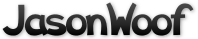Got questions, comments, patches, etc.? Contact Jason Woofenden
diff --git a/draw.h b/draw.h
index d3f9872..d1a2f37 100644 (file)
--- a/draw.h
+++ b/draw.h
@@ -1,6 +1,69 @@
-/*
- * (C)opyright MMVI Anselm R. Garbe <garbeam at gmail dot com>
- */
+
+struct _XCol {
+       unsigned long rgb;
+};
+typedef struct _XCol Col;
+
+struct _XFont {
+       int ascent;
+       int descent;
+       unsigned int h, w;
+       XFontSet set;
+       XFontStruct *xfont;
+};
+typedef struct _XFont Fnt;
+/* X11 types - end */
+
+typedef struct {
+       unsigned int w;
+       unsigned int h;
+       int x;
+       int y;
+       int xOff;
+       int yOff;
+} TextExtents;
+
+
+/* X11 types - begin */
+typedef struct _XDraw Draw;
+struct _XDraw {
+       unsigned int w, h;
+       Display *dpy;
+       int screen;
+       Window win;
+       Drawable drawable;
+       GC gc;
+       Col *fg;
+       Col *bg;
+       Fnt *font;
+};
+
+/* Drawable abstraction */
+Draw *draw_create(Display *dpy, int screen, Window win, unsigned int w, unsigned int h);
+void draw_resize(Draw *draw, unsigned int w, unsigned int h);
+void draw_free(Draw *draw);
+
+/* Fnt abstraction */
+Fnt *font_create(const char *fontname);
+void font_free(Fnt *font);
+
+/* Colour abstraction */
+Col *col_create(const char *colname);
+void col_free(Col *col);
+
+/* Drawing context manipulation */
+void draw_setfont(Draw *draw, Fnt *font);
+void draw_setfg(Draw *draw, Col *col);
+void draw_setbg(Draw *draw, Col *col);
+
+/* Drawing functions */
+void draw_rect(Draw *draw, int x, int y, unsigned int w, unsigned int h);
+void draw_text(Draw *draw, int x, int y, const char *text);
+
+/* Map functions */
+void draw_map(Draw *draw, int x, int y, unsigned int w, unsigned int h);
+
+/* Text functions */
+void draw_getextents(Draw *draw, const char *text, TextExtents *extents);

-extern void error(char *errstr, ...);